# Miscellaneous Exercise Linear Programming Solution - NCERT Class 12

Go back to  'Linear Programming'

## Chapter 12 Ex.12.ME Question 1

Refer to Example 9 (Diet problem)

A dietician has to develop a special diet using two foods P and Q.

Each packet (containing 30 g) of food P contains 12 units of calcium, 4 units of iron, 6 units of cholesterol and 6 units of vitamin A.

Each packet of the same quantity of food Q contains 3 units of calcium, 20 units of iron, 4 units of cholesterol and 3 units of vitamin A. The diet requires at least 240 units of calcium, at least 460 units of iron and at most 300 units of cholesterol.

How many packets of each food should be used to maximize the amount of vitamin A in the diet? What is the maximum amount of vitamin A in the diet?

### Solution

Let the diet contain x and y packets of foods P and Q respectively.

Thus, $$x \ge 0$$ and $$y \ge 0$$

The mathematical formulation of the given problem is as follows.

Maximize

$Z = 6x + 3y\;\;\;\;\;\;\;\;\;\; \ldots \left( 1 \right)$

Subject to the constraints,

\begin{align}4x + y \ge 80\;\;\;\;\;\;\;\;\;\; \ldots \left( 2 \right)\\x + 5y \ge 115\;\;\;\;\;\;\;\;\; \ldots \left( 3 \right)\\3x + 2y \le 150\;\;\;\;\;\;\; \ldots \left( 4 \right)\\x,\;y \ge 0\;\;\;\;\;\;\;\;\;\;\;\;\;\;\; \ldots \left( 5 \right)\end{align}

The feasible region determined by the system of constraints is given by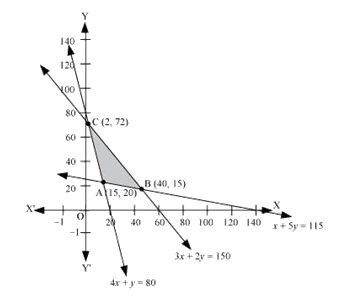The corner points of the feasible region are $$A\left( {15,20} \right),B\left( {40,15} \right)$$ and $$C\left( {2,72} \right)$$.

The values of $$Z$$ at these corner points are as follows:

 Corner point $$Z = 6x + 3y$$ $$\;\;\;{\rm{A}}\left( {15,20} \right)$$ 150 $$\;\;\;{\rm{B}}\left( {40,15} \right)$$ 285 $$\to$$Maximum $$\;\;\;{\rm{C}}\left( {2,72} \right)$$ 228

Thus, the maximum value of Z is 285 at $$B\left( {40,15} \right)$$.

Hence, to maximize the amount of Vitamin A in the diet, 40 packets of Food P and 15 packets of Food Q should be used.

Thus, the maximum amount of Vitamin A in the diet is 285 units.

## Chapter 12 Ex.12.ME Question 2

A farmer mixes two brands P and Q of cattle feed.

Brand P, costing ₹ 250 per bag contains 3 units of nutritional element A, 2.5 units of element B and 2 units of element C.

Brand Q costing ₹ 200 per bag contains 1.5 units of nutritional elements A, 11.25 units of element B, and 3 units of element C.

The minimum requirements of nutrients A, B and C are 18 units, 45 units and 24 units respectively.

Determine the number of bags of each brand which should be mixed in order to produce a mixture having a minimum cost per bag? What is the minimum cost of the mixture per bag?

### Solution

Let the farmer mix x bags of brand P and y bags of brand Q.

The given information can be given in a table as follows:

 Vitamin A (units/kg) Vitamin B (units/kg) Cost (₹/kg) Food P 3 5 60 Food Q 4 2 80 Requirement (units/kg) 8 11

The given problem can be formulated as follows:

Minimize

$Z = 250x + 200y\;\;\;\;\;\;\;\;\;\; \ldots \left( 1 \right)$

Subject to the constraints,

\begin{align}3x + 1.5y \ge 18\;\;\;\;\;\;\;\;\;\;\;\;\;\;\;\; \ldots \left( 2 \right)\\2.5x + 11.25y \ge 45\;\;\;\;\;\;\;\;\;\; \ldots \left( 3 \right)\\2x + 3y \ge 24\;\;\;\;\;\;\;\;\;\;\;\;\;\;\;\;\;\; \ldots \left( 4 \right)\\x,\;y \ge 0\;\;\;\;\;\;\;\;\;\;\;\;\;\;\;\;\;\;\;\;\;\;\;\;\; \ldots \left( 5 \right)\end{align}

The feasible region determined by the system of constraints is given by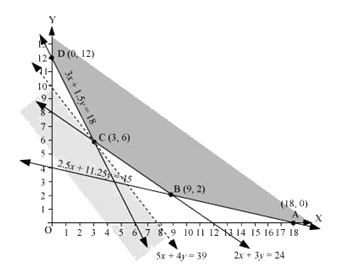The corner points of the feasible region are $$A\left( {18,0} \right),B\left( {9,2} \right),C\left( {3,6} \right)$$ and $$D\left( {0,12} \right)$$.

The values of $$Z$$ at these corner points are as follows:

 Corner point $$Z = 250x + 200y$$ $$\;\;\;{\rm{A}}\left( {18,0} \right)$$ 4500 $$\;\;\;{\rm{B}}\left( {9,2} \right)$$ 2650 $$\;\;\;{\rm{C}}\left( {3,6} \right)$$ 1950 $$\to$$Minimum $$\;\;\;{\rm{D}}\left( {0,12} \right)$$ 2400

As the feasible region is unbounded, therefore, 1950 may or may not be the minimum value of Z.

For this, we draw a graph of the inequality,$$250x + 200y < 1950$$ or $$5x + 4y < 39$$, and check whether the resulting half plane has points in common with the feasible region or not.

Since, the feasible region has no common point with $$5x + 4y < 39$$.

Thus, the minimum value of Z is 1950 at $$C\left( {3,6} \right)$$.

Therefore, 3 bags of brand P and 6 bags of brand Q should be used in the mixture to minimize the cost to ₹ 1950.

## Chapter 12 Ex.12.ME Question 3

A dietician wishes to mix together two kinds of food X and Y in such a way that the mixture contains at least 10 units of vitamin A, 12 units of vitamin B and 8 units of vitamin C. The vitamin content of one kg food is given below:

 Food Vitamin A Vitamin B Vitamin C X 1 2 3 Y 2 2 1

One kg of food X costs ₹ 16 and one kg of food Y costs ₹ 20. Find the least cost of the mixture which will produce the required diet?

### Solution

Let the mixture contain x kg of food X and y kg of food Y.

The mathematical formulation of the given problem is as follows:

Minimize

$Z = 16x + 20y\;\;\;\;\;\;\;\;\;\; \ldots \left( 1 \right)$

Subject to the constraints,

\begin{align}x + 2y \ge 10\;\;\;\;\;\;\;\;\;\; \ldots \left( 2 \right)\\x + y \ge 6\;\;\;\;\;\;\;\;\;\;\;\;\; \ldots \left( 3 \right)\\3x + y \ge 8\;\;\;\;\;\;\;\;\;\;\; \ldots \left( 4 \right)\\x,\;y \ge 0\;\;\;\;\;\;\;\;\;\;\;\;\;\; \ldots \left( 5 \right)\end{align}

The feasible region determined by the system of constraints is given by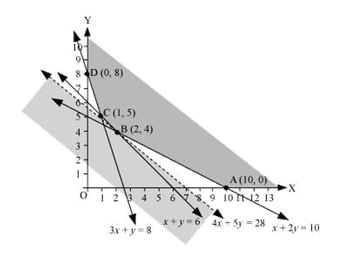The corner points of the feasible region are $$A\left( {10,0} \right),B\left( {2,4} \right),C\left( {1,5} \right)$$ and $$D\left( {0,8} \right)$$.

The values of Z at these corner points are as follows:

 Corner point $$Z = 16x + 20y$$ $$\;\;\;\;\;{\rm{A}}\left( {10,0} \right)$$ 160 $$\;\;\;\;\;{\rm{B}}\left( {2,4} \right)$$ 112 $$\to$$Minimum $$\;\;\;\;\;{\rm{C}}\left( {1,5} \right)$$ 116 $$\;\;\;\;\;{\rm{D}}\left( {0,8} \right)$$ 160

As the feasible region is unbounded, therefore, 112 may or may not be the minimum value of Z.

For this, we draw a graph of the inequality, $$16x + 20y < 112$$ or $$4x + 5y < 28$$, and check whether the resulting half plane has points in common with the feasible region or not.

Since, the feasible region has no common point with $$4x + 5y < 28$$.

Thus, the minimum value of Z is 112 at $$B\left( {2,4} \right)$$.

Therefore, the mixture should contain 2 kg of food X and 4 kg of food Y. The minimum cost of the mixture is ₹ 112.

## Chapter 12 Ex.12.ME Question 4

A manufacturer makes two types of toys A and B. Three machines are needed for this purpose and the time (in minutes) required for each toy on the machines is given below:

 Types of Toys Machines I II III A 12 8 6 B 6 0 9

Each machine is available for a maximum of 6 hours per day. If the profit on each toy of type A is ₹ 7.50 and that on each toy of type B is ₹ 5, show that 15 toys of type A and 30 of type B should be manufactured in a day to get maximum profit.

### Solution

Let x and y toys of type A and type B respectively be manufactured in a day.

The given problem can be formulated as follows:

Maximize

$Z = 7.5x + 5y\;\;\;\;\;\;\;\;\;\; \ldots \left( 1 \right)$

Subject to the constraints,

\begin{align}2x + y \le 60\;\;\;\;\;\;\;\;\;\;\;\;\;\; \ldots \left( 2 \right)\\x \le 20\;\;\;\;\;\;\;\;\;\;\;\;\;\;\;\;\;\;\;\;\; \ldots \left( 3 \right)\\2x + 3y \le 8\;\;\;\;\;\;\;\;\;\;\;\;\;\; \ldots \left( 4 \right)\\x,\;y \ge 0\;\;\;\;\;\;\;\;\;\;\;\;\;\;\;\;\;\;\; \ldots \left( 5 \right)\end{align}

The feasible region determined by the constraints is given by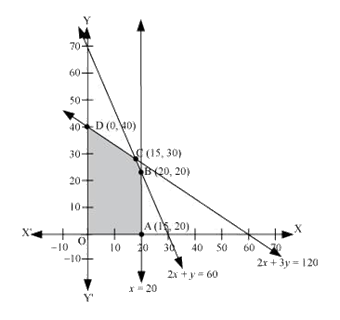The corner points of the feasible region are $$A\left( {20,0} \right),B\left( {20,20} \right),C\left( {15,30} \right)$$ and $$D\left( {0,40} \right)$$.

The values of Z at these corner points are as follows:

 Corner point $$Z = 7.5x + 5y$$ $$\;\;\;{\rm{A}}\left( {20,0} \right)$$ 150 $$\;\;\;{\rm{B}}\left( {20,20} \right)$$ 250 $$\;\;\;{\rm{C}}\left( {15,30} \right)$$ 262.5 $$\to$$Maximum $$\;\;\;{\rm{D}}\left( {0,40} \right)$$ 200

Thus, the maximum value of Z is 262.5 at $$C\left( {15,30} \right)$$.

Hence, the manufacturer should manufacture 15 toys of type A and 30 toys of type B to maximize the profit.

## Chapter 12 Ex.12.ME Question 5

An aeroplane can carry a maximum of 200 passengers. A profit of ₹ 1000 is made on each executive class ticket and a profit of ₹ 600 is made on each economy class ticket. The airline reserves at least 20 seats for executive class. However, at least 4 times as many passengers prefer to travel by economy class than by the executive class.

Determine how many tickets of each type must be sold in order to maximize the profit for the airline. What is the maximum profit?

### Solution

Let the airline sell x tickets of executive class and y tickets of economy class.

The mathematical formulation of the given problem is as follows.

Maximize

$Z = 1000x + 600y\;\;\;\;\;\;\;\;\; \ldots \left( 1 \right)$

Subject to the constraints,

\begin{align}x + y \le 200\;\;\;\;\;\;\;\;\; \ldots \left( 2 \right)\\x \ge 20\;\;\;\;\;\;\;\;\;\;\;\;\;\;\;\; \ldots \left( 3 \right)\\y - 4x \ge 0\;\;\;\;\;\;\;\;\;\;\; \ldots \left( 4 \right)\\x,\;y \ge 0\;\;\;\;\;\;\;\;\;\;\;\;\;\; \ldots \left( 5 \right)\end{align}

The feasible region determined by the constraints is given by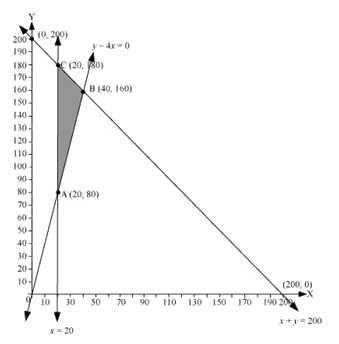The corner points of the feasible region are $$A\left( {20,80} \right),B\left( {40,160} \right)$$and $$C\left( {20,180} \right)$$.

The values of Z at these corner points are as follows:

 Corner point $$Z = 1000x + 600y$$ $$\;\;\;{\rm{A}}\left( {20,80} \right)$$ 68000 $$\;\;\;{\rm{B}}\left( {40,160} \right)$$ 136000 $$\to$$Maximum $$\;\;\;{\rm{C}}\left( {20,180} \right)$$ 128000

Thus, the maximum value of Z is 136000 at $$B\left( {40,160} \right)$$.

Therefore, 40 tickets of executive class and 160 tickets of economy class should be sold to maximize the profit and the maximum profit is ₹ 136000.

## Chapter 12 Ex.12.ME Question 6

Two godowns A and B have grain capacity of 100 quintals and 50 quintals respectively. They supply to 3 ration shops, D, E and F whose requirements are 60, 50 and 40 quintals respectively. The cost of transportation per quintal from the godowns to the shops are given in the following table:

 Transportation cost per quintal (₹/kg) From/To A B D           E           F 6        3     2.50 4        2        3

How should the supplies be transported in order that the transportation cost is minimum? What is the minimum cost?

### Solution

Let godown A supply x and y quintals of grain to the shops D and E, respectively.

Then, $$\left( {100 - x - y} \right)$$ will be supplied to shop F.

The requirement at shop D is 60 quintals since x quintals are transported from godown A. Therefore, the remaining $$\left( {60 - x} \right)$$ quintals will be transported from godown B.

Similarly, $$\left( {50 - y} \right)$$ quintals and $$40 - \left( {100 - x - y} \right) = \left( {x + y - 60} \right)$$ quintals will be transported from godown B to shop E and F respectively.

The given problem can be represented diagrammatically as follows: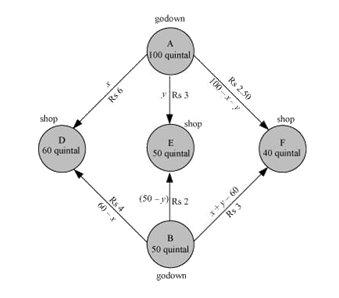Thus,

$$\qquad\qquad x \ge 0,\;y \ge 0$$ and $$100 - x - y \ge 0$$

$$\qquad\qquad \Rightarrow x \ge 0,\;y \ge 0$$ and $$x + y \le 100$$

And

$$\qquad\qquad 60 - x \ge 0,\;50 - y \ge 0$$ and $$x + y - 60 \ge 0$$

$$\qquad\qquad \Rightarrow x \le 60,\;y \le 50$$ and $$x + y \ge 60$$

Total transportation cost Z is given by

\begin{align}Z &= 6x + 3y + 2.5\left( {100 - x - y} \right) \\&\quad+ 4\left( {60 - x} \right) + 2\left( {50 - y} \right) + 3\left( {x + y - 60} \right)\\ &= 6x + 3y + 250 - 2.5x - 2.5y + 240 - 4x \\&\quad+ 100 - 2y + 3x + 3y - 180\\ &= 2.5x + 1.5y + 410\end{align}

The given problem can be formulated as

Minimize

$Z = 2.5x + 1.5y + 410\;\;\;\;\;\;\;\;\; \ldots \left( 1 \right)$

Subject to the constraints,

\begin{align}x + y \le 100\;\;\;\;\;\;\;\;\; \ldots \left( 2 \right)\\x \le 60\;\;\;\;\;\;\;\;\;\;\;\;\;\;\;\; \ldots \left( 3 \right)\\y \le 50\;\;\;\;\;\;\;\;\;\;\;\;\;\;\;\; \ldots \left( 4 \right)\\x + y \ge 60\;\;\;\;\;\;\;\;\;\;\; \ldots \left( 5 \right)\\x,\;y \ge 0\;\;\;\;\;\;\;\;\;\;\;\;\;\; \ldots \left( 6 \right)\end{align}

The feasible region determined by the system of constraints is given by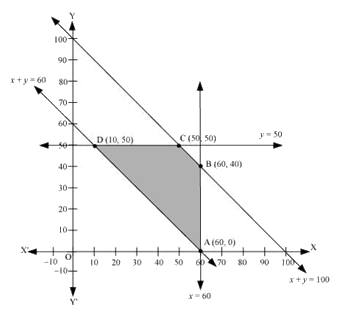The corner points are $$A\left( {60,0} \right),B\left( {60,40} \right),C\left( {50,50} \right)$$ and $$D\left( {10,50} \right)$$.

The values of $$Z$$ at these corner points are as follows:

 Corner point $$Z = 2.5x + 1.5y + 410$$ $$\;\;\;{\rm{A}}\left( {60,0} \right)$$ $$560$$ $$\;\;\;{\rm{B}}\left( {60,40} \right)$$ $$620$$ $$\;\;\;{\rm{C}}\left( {50,50} \right)$$ $$610$$ $$\;\;\;{\rm{D}}\left( {10,50} \right)$$ $$510$$ $$\to$$Minimum

Thus, the minimum value of $$Z$$ is $$510$$ at $$D\left( {10,50} \right)$$.

Thus, the minimum cost is ₹ $$510.$$

## Chapter 12 Ex.12.ME Question 7

An oil company has two depots A and B with capacities of 7000 L and 4000 L respectively. The company is to supply oil to three petrol pumps, D, E and F whose requirements are 4500L, 3000L and 3500L respectively. The distance (in km) between the depots and the petrol pumps is given in the following table:

 Distance (in km) From/To A B D       E       F 7     6     3 3     4     2

Assuming that the transportation cost of 10 litres of oil is ₹ 1 per km, how should the delivery be scheduled in order that the transportation cost is minimum? What is the minimum cost?

### Solution

Let x and y litres of oil be supplied from A to the petrol pumps, D and E. Then,$$\left( {7000 - x - y} \right)$$ will be supplied from A to petrol pump F.

The requirement at petrol pump D is 4500 L. Since x L are transported from depot A, the remaining $$\left( {4500 - x} \right)$$L will be transported from petrol pump B.

Similarly,$$\left( {3000 - y} \right)$$L and $$3500 - \left( {7000 - x - y} \right) = \left( {x + y - 3500} \right)$$L will be transported from depot B to petrol pump E and F respectively.

The given problem can be represented diagrammatically as follows: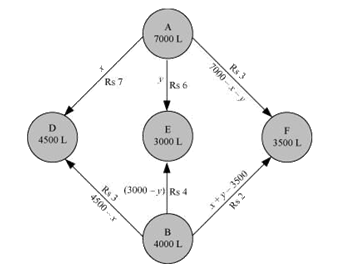Thus,

$$\qquad\qquad x \ge 0,\;y \ge 0$$ and $$7000 - x - y \ge 0$$

$$\qquad\qquad \Rightarrow x \ge 0,\;y \ge 0$$ and $$x + y \le 7000$$

And

$$\qquad\qquad 4500 - x \ge 0,\;3000 - y \ge 0$$ and $$x + y - 3500 \ge 0$$

$$\qquad\qquad \Rightarrow x \le 4500,\;y \le 3000$$ and $$x + y \ge 3500$$

Cost of transporting 10 L of petrol in ₹ $$= 1$$

Cost of transporting 1 L of petrol in ₹ $$= \frac{1}{{10}}$$

Therefore, total transportation cost is given by,

\begin{align}Z &= \frac{7}{{10}} \times x + \frac{6}{{10}}y + \frac{3}{{10}}\left( {7000 - x - y} \right) + \frac{3}{{10}}\left( {4500 - x} \right) \\&\quad+ \frac{4}{{10}}\left( {3000 - y} \right) + \frac{2}{{10}}\left( {x + y - 3500} \right)\\ &= 0.3x + 0.1y + 3950\end{align}

The problem can be formulated as follows.

Minimize

$Z = 0.3x + 0.1y + 3950{\rm{ }}......\left( 1 \right)$

Subject to the constraints,

\begin{align} & x + y \le 7000{\rm{ }}\quad\quad\quad\;\;......\left( 2 \right)\\& \quad x \le 4500{\rm{ }}\quad\quad\quad\;\;\;\;\;......\left( 3 \right)\\&\quad y \le 3000 \quad\quad\quad\;\;\;\;\;......\left( 4 \right)\\&x + y \ge 3500 \quad\quad\quad\;\;......\left( 5 \right)\\&\quad x,\;y \ge 0{\rm{ }}\quad\quad\quad\;\;\;\;\;\;......\left( 6 \right)\end{align}

The feasible region determined by the constraints is given by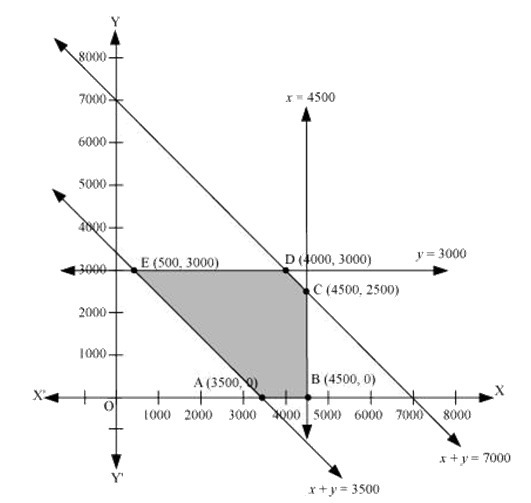The corner points of the feasible region are $$A\left( {3500,0} \right),B\left( {4500,0} \right),C\left( {4500,2500} \right),$$$$D\left( {4000,3000} \right)$$ and $$E\left( {500,3000} \right)$$.

The values of Z at these corner points are as follows:

 Corner point $$Z = 0.3x + 0.1y + 3950$$ $$\;\;\;{\rm{A}}\left( {3500,0} \right)$$ $$5000$$ $$\;\;\;{\rm{B}}\left( {4500,0} \right)$$ $$5300$$ $$\;\;\;{\rm{C}}\left( {4500,2500} \right)$$ $$5550$$ $$\;\;\;{\rm{D}}\left( {4000,3000} \right)$$ $$5450$$ $$\;\;\;{\rm{E}}\left( {500,3000} \right)$$ $$4400$$ $$\to$$Minimum

Thus, the minimum value of $$Z$$ is $$4400$$ at $$E\left( {500,3000} \right)$$.

Therefore, the minimum transportation cost is ₹ $$4400$$.

## Chapter 12 Ex.12.ME Question 8

A fruit grower can use two types of fertilizer in his garden, brand P and brand Q. The amounts (in kg) of nitrogen, phosphoric acid, potash, and chlorine in a bag of each brand are given in the table. Tests indicate that the garden needs at least 240 kg of phosphoric acid at least 270 kg of potash and at most 310 kg of chlorine.

If the grower wants to minimize the amount of nitrogen added to the garden, how many bags of each brand should be used? What is the minimum amount of nitrogen added in the garden?

 Kg per bag Brand P Brand Q Nitrogen Phosphoric Acid       Potash      Chlorine 3      1      3    1.5 3.5      2     1.5      2

### Solution

Let the fruit grower use x bags of brand P and y bags of brand Q.

The problem can be formulated as follows.

Minimize

$Z = 3x + 3.5y\;\;\;\;\;\;\;\;\;\; \ldots \left( 1 \right)$

Subject to the constraints,

\begin{align}x + 2y \ge 240\;\;\;\;\;\;\;\;\;\; \ldots \left( 2 \right)\\x + 0.5y \ge 90\;\;\;\;\;\;\;\;\; \ldots \left( 3 \right)\\1.5x + 2y \le 310\;\;\;\;\;\; \ldots \left( 4 \right)\\x,\;y \ge 0\;\;\;\;\;\;\;\;\;\;\;\;\;\;\;\;\; \ldots \left( 5 \right)\end{align}

The feasible region determined by the system of constraints is given by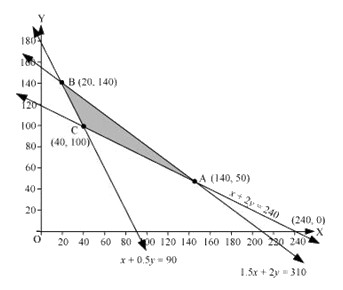The corner points are $$A\left( {140,50} \right),B\left( {20,140} \right)$$ and $$C\left( {40,100} \right)$$.

The values of Z at these corner points are as follows:

 Corner point $$Z = 3x + 3.5y$$ $$\;\;\;{\rm{A}}\left( {140,50} \right)$$ 595 $$\;\;\;{\rm{B}}\left( {20,140} \right)$$ 550 $$\;\;\;{\rm{C}}\left( {40,100} \right)$$ 470 $$\to$$Minimum

Thus, the minimum value of Z is 470 at $$C\left( {40,100} \right)$$.

Therefore, 40 bags of brand P and 100 bags of brand Q should be added to the garden to minimize the amount of nitrogen.

Hence, the minimum amount of nitrogen added to the garden is 470 kg.

## Chapter 12 Ex.12.ME Question 9

Refer to question 8. If the grower wants to maximize the amount of nitrogen added to the garden, how many bags of each brand should be added? What is the maximum amount of nitrogen added?

### Solution

Let the fruit grower use x bags of brand P and y bags of brand Q.

The problem can be formulated as follows.

Maximize

$Z = 3x + 3.5y\;\;\;\;\;\;\;\;\;\; \ldots \left( 1 \right)$

Subject to the constraints,

\begin{align}x + 2y \ge 240\;\;\;\;\;\;\;\;\;\; \ldots \left( 2 \right)\\x + 0.5y \ge 90\;\;\;\;\;\;\;\;\; \ldots \left( 3 \right)\\1.5x + 2y \le 310\;\;\;\;\;\; \ldots \left( 4 \right)\\x,\;y \ge 0\;\;\;\;\;\;\;\;\;\;\;\;\;\;\;\;\; \ldots \left( 5 \right)\end{align}

The feasible region determined by the system of constraints is given by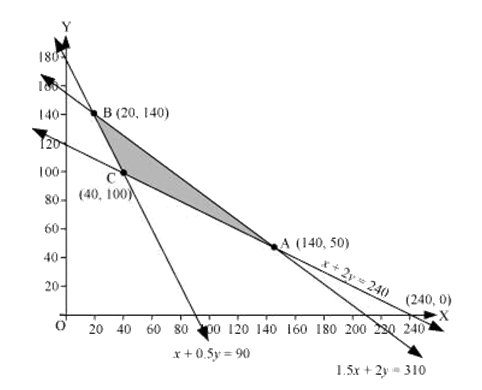The corner points are $$A\left( {140,50} \right),B\left( {20,140} \right)$$ and $$C\left( {40,100} \right)$$.

The values of Z at these corner points are as follows:

 Corner point $$Z = 3x + 3.5y$$ $$\;\;\;{\rm{A}}\left( {140,50} \right)$$ 595 $$\to$$Maximum $$\;\;\;{\rm{B}}\left( {20,140} \right)$$ 550 $$\;\;\;{\rm{C}}\left( {40,100} \right)$$ 470

Thus, the maximum value of Z is 595 at $$A\left( {140,50} \right)$$.

Hence, 140 bags of brand P and 50 bags of brand Q should be used to maximize the amount of nitrogen.

Thus, the maximum amount of nitrogen added to the garden is 595 kg.

## Chapter 12 Ex.12.ME Question 10

A toy company manufactures two types of dolls, $$A$$ and $$B$$. Market tests and available resources have indicated that the combined production level should not exceed $$1200$$ dolls per week and the demand for dolls of type $$B$$ is at most half of that for dolls of type $$A$$. Further, the production level of dolls of type $$A$$ can exceed three times the production of dolls of other type by at most $$600$$ units.

If the company makes profit of ₹ $$12$$ and ₹ $$16$$ per doll respectively on dolls $$A$$ and $$B$$, how many of each should be produced weekly in order to maximize the profit?

### Solution

Let $$x$$ and $$y$$ be the number of dolls of type $$A$$ and $$B$$ respectively that are produced per week.

The given problem can be formulated as follows.

Maximize

$Z = 12x + 16y\;\;\;\;\;\;\;\;\; \ldots \left( 1 \right)$

Subject to the constraints,

\begin{align}x + y \le 1200\;\;\;\;\;\;\;\;\;\;\;\;\;\; \ldots \left( 2 \right)\\y \le \frac{x}{2} \Rightarrow x \ge 2y\;\;\;\;\;\;\;\;\; \ldots \left( 3 \right)\\x - 3y \le 600\;\;\;\;\;\;\;\;\;\;\;\;\;\; \ldots \left( 4 \right)\\x,\;y \ge 0\;\;\;\;\;\;\;\;\;\;\;\;\;\;\;\;\;\;\;\;\; \ldots \left( 5 \right)\end{align}

The feasible region determined by the system of constraints is given by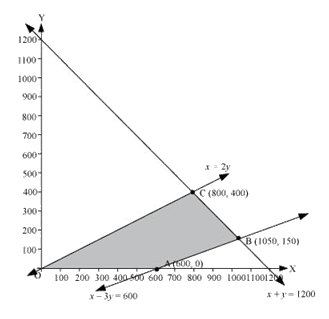The corner points are $$A\left( {600,0} \right),B\left( {1050,150} \right)$$ and $$C\left( {800,400} \right)$$.

The values of Z at these corner points are as follows:

 Corner point $$Z = 12x + 16y$$ $$\;\;\;{\rm{A}}\left( {600,0} \right)$$ $$7200$$ $$\;\;\;{\rm{B}}\left( {1050,150} \right)$$ $$15000$$ $$\;\;\;{\rm{C}}\left( {800,400} \right)$$ $$16000$$ $$\to$$Maximum

Thus, the maximum value of $$Z$$ is $$16000$$ at $$\left( {800,400} \right)$$.

Hence, $$800$$ and $$400$$ dolls of type $$A$$ and type $$B$$ should be produced respectively to get the maximum profit of ₹$$16000$$.

Related Sections
Related Sections
Instant doubt clearing with Cuemath Advanced Math Program